International
Tables for
Crystallography
Volume B
Reciprocal space
Edited by U. Shmueli

International Tables for Crystallography (2006). Vol. B, ch. 1.3, p. 27   | 1 | 2 |

Section 1.3.2.2.4. Integration,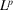spaces

G. Bricognea

aMRC Laboratory of Molecular Biology, Hills Road, Cambridge CB2 2QH, England, and LURE, Bâtiment 209D, Université Paris-Sud, 91405 Orsay, France

1.3.2.2.4. Integration,spaces

| top | pdf |

The Riemann integral used in elementary calculus suffers from the drawback that vector spaces of Riemann-integrable functions over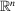are not complete for the topology of convergence in the mean: a Cauchy sequence of integrable functions may converge to a non-integrable function.

To obtain the property of completeness, which is fundamental in functional analysis, it was necessary to extend the notion of integral. This was accomplished by Lebesgue [see Berberian (1962), Dieudonné (1970), or Chapter 1 of Dym & McKean (1972)and the references therein, or Chapter 9 of Sprecher (1970)], and entailed identifying functions which differed only on a subset of zero measure in(such functions are said to be equal almost everywhere'). The vector spaces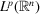consisting of function classes f modulo this identification for which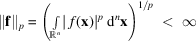are then complete for the topology induced by the norm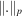: the limit of every Cauchy sequence of functions inis itself a function in(Riesz–Fischer theorem).

The space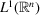consists of those function classes f such that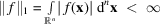which are called summable or absolutely integrable. The convolution product: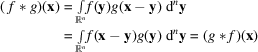is well defined; combined with the vector space structure of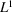, it makesinto a (commutative) convolution algebra. However, this algebra has no unit element: there is no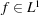such that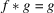for all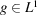; it has only approximate units, i.e. sequences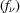such that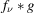tends to g in thetopology as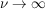. This is one of the starting points of distribution theory.

The space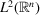of square-integrable functions can be endowed with a scalar product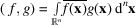which makes it into a Hilbert space. The Cauchy–Schwarz inequality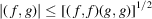generalizes the fact that the absolute value of the cosine of an angle is less than or equal to 1.

The space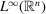is defined as the space of functions f such that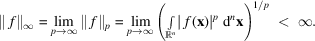The quantity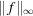is called the essential sup norm' of f, as it is the smallest positive number which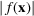exceeds only on a subset of zero measure in. A function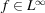is called essentially bounded.

References

Berberian, S. K. (1962). Measure and integration. New York: Macmillan. [Reprinted by Chelsea, New York, 1965.]
Dieudonné, J. (1970). Treatise on analysis, Vol. II. New York and London: Academic Press.
Dym, H. & McKean, H. P. (1972). Fourier series and integrals. New York and London: Academic Press.
Sprecher, D. A. (1970). Elements of real analysis. New York: Academic Press. [Reprinted by Dover Publications, New York, 1987.]